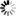Welcome to Central Library, SUST
Normal view

# Computer Algebra in Scientific Computing [electronic resource] : 15th International Workshop, CASC 2013, Berlin, Germany, September 9-13, 2013. Proceedings / edited by Vladimir P. Gerdt, Wolfram Koepf, Ernst W. Mayr, Evgenii V. Vorozhtsov.

Material type:TextSeries: Lecture Notes in Computer Science ; 8136Publisher: Cham : Springer International Publishing : Imprint: Springer, 2013Description: XVI, 443 p. 58 illus. online resourceContent type:
• text
Media type:
• computer
Carrier type:
• online resource
ISBN:
• 9783319022970
Additional physical formats: Printed edition:: No titleDDC classification:
• 005.1 23
LOC classification:
• QA76.9.A43
Online resources:
Contents:
Polynomial algebra -- the solution of tropical linear systems and tropical polynomial systems -- the theory of matrices -- the use of computer algebra for the investigation of various mathematical and applied topics related to ordinary differential equations -- applications of symbolic computations for solving partial differential equations in mathematical physics -- problems arising at the application of computer algebra methods for finding infinitesimal symmetries -- applications of symbolic and symbolic-numeric algorithms in mechanics and physics -- automatic differentiation -- the application of the CAS Mathematica for the simulation of quantum error correction in quantum computing -- the application of the CAS GAP for the enumeration of Schur rings over the group A5 -- constructive computation of zero separation bounds for arithmetic expressions -- the parallel implementation of fast Fourier transforms with the aid of the Spiral library generation system -- the use of object-oriented languages such as Java or Scala for implementation of categories as type classes -- a survey of industrial applications of approximate computer algebra.
Summary: This book constitutes the proceedings of the 14th International Workshop on Computer Algebra in Scientific Computing, CASC 2013, held in Berlin, Germany, in September 2013. The 33 full papers presented were carefully reviewed and selected for inclusion in this book. The papers address issues such as polynomial algebra; the solution of tropical linear systems and tropical polynomial systems; the theory of matrices; the use of computer algebra for the investigation of various mathematical and applied topics related to ordinary differential equations (ODEs); applications of symbolic computations for solving partial differential equations (PDEs) in mathematical physics; problems arising at the application of computer algebra methods for finding infinitesimal symmetries; applications of symbolic and symbolic-numeric algorithms in mechanics and physics; automatic differentiation; the application of the CAS Mathematica for the simulation of quantum error correction in quantum computing; the application of the CAS GAP for the enumeration of Schur rings over the group A5; constructive computation of zero separation bounds for arithmetic expressions; the parallel implementation of fast Fourier transforms with the aid of the Spiral library generation system; the use of object-oriented languages such as Java or Scala for implementation of categories as type classes; a survey of industrial applications of approximate computer algebra.
Tags from this library: No tags from this library for this title.
Star ratingsAverage rating: 0.0 (0 votes)
No physical items for this record

Polynomial algebra -- the solution of tropical linear systems and tropical polynomial systems -- the theory of matrices -- the use of computer algebra for the investigation of various mathematical and applied topics related to ordinary differential equations -- applications of symbolic computations for solving partial differential equations in mathematical physics -- problems arising at the application of computer algebra methods for finding infinitesimal symmetries -- applications of symbolic and symbolic-numeric algorithms in mechanics and physics -- automatic differentiation -- the application of the CAS Mathematica for the simulation of quantum error correction in quantum computing -- the application of the CAS GAP for the enumeration of Schur rings over the group A5 -- constructive computation of zero separation bounds for arithmetic expressions -- the parallel implementation of fast Fourier transforms with the aid of the Spiral library generation system -- the use of object-oriented languages such as Java or Scala for implementation of categories as type classes -- a survey of industrial applications of approximate computer algebra.

This book constitutes the proceedings of the 14th International Workshop on Computer Algebra in Scientific Computing, CASC 2013, held in Berlin, Germany, in September 2013. The 33 full papers presented were carefully reviewed and selected for inclusion in this book. The papers address issues such as polynomial algebra; the solution of tropical linear systems and tropical polynomial systems; the theory of matrices; the use of computer algebra for the investigation of various mathematical and applied topics related to ordinary differential equations (ODEs); applications of symbolic computations for solving partial differential equations (PDEs) in mathematical physics; problems arising at the application of computer algebra methods for finding infinitesimal symmetries; applications of symbolic and symbolic-numeric algorithms in mechanics and physics; automatic differentiation; the application of the CAS Mathematica for the simulation of quantum error correction in quantum computing; the application of the CAS GAP for the enumeration of Schur rings over the group A5; constructive computation of zero separation bounds for arithmetic expressions; the parallel implementation of fast Fourier transforms with the aid of the Spiral library generation system; the use of object-oriented languages such as Java or Scala for implementation of categories as type classes; a survey of industrial applications of approximate computer algebra.

There are no comments on this title.